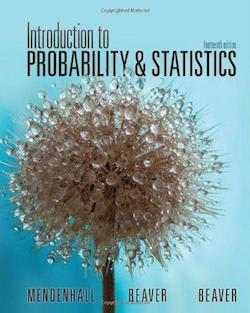Statistics Introduction to Probability and Statistics# Introduction to Probability and Statistics Mendenhall • 14th Edition • 978-1133103752

Not the textbook you were looking for? Pick another one here.

## Ch.1 - Describing Data with Graphs

Qualitative Data Quantitative Data

## Ch. 2 - Describing Data with Numerical Measures

Measures of Central Tendency Measures of Spread: Standard Deviation Measures of Spread: Interquartile Range Boxplots (The Five Number Summary)

## Ch. 4 - Probability and Probability Distributions

Probability Notations Addition Rule Multiplication Rule Discrete Random Variable Discrete Random Variable Mean and Standard Deviation

## Ch. 5 - Several Useful Discrete Distributions

The Binomial Distribution The Binomial Formula The Binomial Table

## Ch. 6 - The Normal Probability Distribution

The Uniform Distribution The Normal Distribution The Z-Score The Z-Table Probabilities from Scores Scores from Probability

## Ch. 7 - Sampling Distributions

Sampling Distribution for a Sample Mean Sampling Distribution for a Sample Proportion

## Ch. 8 - Large-Sample Estimation

Large Sample Confidence Interval for a Population Mean Confidence Interval for a Population Proportion Sample Size

## Ch. 9 - Large-Sample Tests of Hypotheses

The Idea Behind Hypothesis Testing Type 1 & Type 2 Errors Null and Alternative Hypotheses Critical Values & Rejection Regions Large Sample Test Statistic: Mean Test Statistic: Proportion

## Ch. 10 - Inference from Small Samples

Small Sample Confidence Interval for a Population Mean Small Sample Test Statistic: Mean